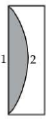Ray optics
Question

# A piano convex lens fits exactly into a piano concave lens. Their plane surfaces are parallel to each other. If lenses are made of different materials of refractive indices ${\mu }_{1}$ and ${\mu }_{2}$ and R is the radius of curvature of the curved surface of the lenses, then the focal length of the combination is

Moderate
Solution

## The combination of two lenses 1 and 2 is as shown in figure.$\therefore \text{\hspace{0.17em}\hspace{0.17em}\hspace{0.17em}}\frac{1}{f}=\frac{1}{{f}_{1}}+\frac{1}{{f}_{2}}$According to lens maker's formula

Get Instant Solutions